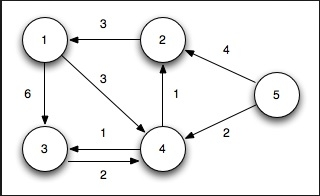# Single-Source Shortest Paths, Nonnegative Weights

Data StructureAnalysis of AlgorithmsAlgorithms

The single source shortest path algorithm (for non-negative weight) is also known Dijkstra algorithm. There is a given graph G(V,E) with its adjacency matrix representation, and a source vertex is also provided. Dijkstra’s algorithm to find the minimum shortest path between source vertex to any other vertex of the graph G.From starting node to any other node, find the smallest distances. In this problem the graph is represented using the adjacency matrix. (Cost matrix and adjacency matrix is similar for this purpose).

Input − The adjacency matrix −

0 3 6 ∞ ∞ ∞ ∞
3 0 2 1 ∞ ∞ ∞
6 2 0 1 4 2 ∞
∞ 1 1 0 2 ∞ 4
∞ ∞ 4 2 0 2 1
∞ ∞ 2 ∞ 2 0 1
∞ ∞ ∞ 4 1 1 0

Output

0 to 1, Using: 0, Cost: 3

0 to 2, Using: 1, Cost: 5

0 to 3, Using: 1, Cost: 4

0 to 4, Using: 3, Cost: 6

0 to 5, Using: 2, Cost: 7

0 to 6, Using: 4, Cost: 7

## Algorithm

### dijkstraShortestPath(n, dist, next, start)

Input − Total number of nodes n, distance list for each vertex, next list to store which node comes next, and the seed or start vertex.

Output − The shortest paths from start to all other vertices.

Begin
create a status list to hold the current status of the selected node
for all vertices u in V do
status[u] := unconsidered
dist[u] := distance from source using cost matrix
next[u] := start
done
status[start] := considered, dist[start] := 0 and next[start] := φ
while take unconsidered vertex u as distance is minimum do
status[u] := considered
for all vertex v in V do
if status[v] = unconsidered then
if dist[v] > dist[u] + cost[u,v] then
dist[v] := dist[u] + cost[u,v]
next[v] := u
done
done
End

## Example(C++)

#include<iostream>
#define V 7
#define INF 999
using namespace std;
//Cost matrix of the graph
int costMat[V][V] = {
{0, 3, 6, INF, INF, INF, INF},
{3, 0, 2, 1, INF, INF, INF},
{6, 2, 0, 1, 4, 2, INF},
{INF, 1, 1, 0, 2, INF, 4},
{INF, INF, 4, 2, 0, 2, 1},
{INF, INF, 2, INF, 2, 0, 1},
{INF, INF, INF, 4, 1, 1, 0}
};
int minimum(int *status, int *dis, int n){
int i, min, index;
min = INF;
for(i = 0; i<n; i++)
if(dis[i] < min && status[i] == 1){
min = dis[i];
index = i;
}
if(status[index] == 1)
return index;//minimum unconsidered vertex distance
else
return -1;//when all vertices considered
}
void dijkstra(int n, int *dist,int *next, int s){
int status[V];
int u, v;
//initialization
for(u = 0; u<n; u++){
status[u] = 1;//unconsidered vertex
dist[u] = costMat[u][s];//distance from source
next[u] = s;
}
//for source vertex
status[s] = 2; dist[s] = 0; next[s] = -1;//-1 for starting vertex
while((u = minimum(status, dist, n)) > -1){
status[u] = 2;//now considered
for(v = 0; v<n; v++)
if(status[v] == 1)
if(dist[v] > dist[u] + costMat[u][v]){
dist[v] = dist[u] + costMat[u][v];//update distance
next[v] = u;
}
}
}
main(){
int dis[V], next[V], i, start = 0;
dijkstra(V, dis, next, start);
for(i = 0; i<V; i++)
if(i != start)
cout << start << " to " << i <<", Using: " << next[i] << ", Cost: " << dis[i] << endl;
}

## Output

0 to 1, Using: 0, Cost: 3
0 to 2, Using: 1, Cost: 5
0 to 3, Using: 1, Cost: 4
0 to 4, Using: 3, Cost: 6
0 to 5, Using: 2, Cost: 7
0 to 6, Using: 4, Cost: 7